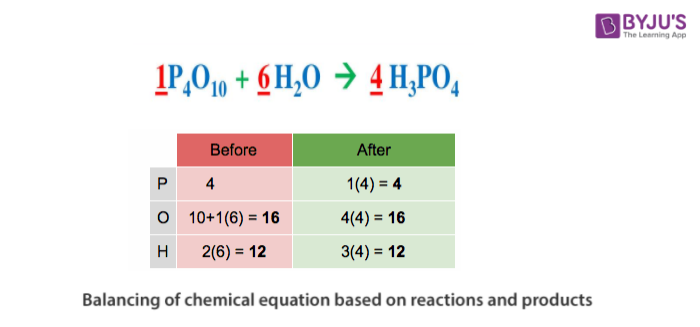# Balancing Of Chemical Equation Based On Reactants And Products

## Balanced chemical equation

The representation of a chemical reaction in the form of symbols (substances) is known as chemical equation. A chemical equation consists of reactants, products and an arrow showing the direction of reaction. The equation in which number of atoms of all the molecules is equal on both sides of the equation is known as balanced chemical equation.Law of conservation of mass governs the balancing of a chemical equation. According to this law, mass can neither be created nor be destroyed in a chemical reaction and obeying this law total mass of the elements or molecules present on the reactant side should be equal to the total mass of elements or molecules present on the product side. If the number of atoms of the elements/molecules on the reactant side are not equal to the number of atoms of the elements/molecules on the product side, then it is said to be an unbalanced chemical equation. For example:

$$\begin{array}{l}Fe~ +~ H_2O \rightarrow Fe_3O_4~ +~ H_2\end{array}$$

In the above example, the law of conservation of mass is not applied. The number of iron atoms on the left side is not equal to the number of iron atoms on the right side; there is one iron atom on the left side whereas there are three iron atoms on the right side of the equation.

Unbalanced equation can be represented in given table below:

 Element No of atoms on reactant side No of atoms on product side Fe(Iron) 1 3 H(Hydrogen) 2 2 O(Oxygen) 1 4

Now, we need to balance the above chemical reaction.

We will first take the maximum number of atoms present on either side of the reaction, that we can find on product side. (Oxygen = 4).

Then we multiply the number of oxygen atoms on reactant side by 4, such that the number of oxygen atoms on both sides of the reaction is balanced.

The equation becomes

$$\begin{array}{l}Fe~ +4~ H_2O \rightarrow Fe_3O_4 ~+~ H_2\end{array}$$

Now the number of hydrogen atoms is 8 on the reactant side which is more than that of the product side, hence we need to balance hydrogen atoms, and hence we multiply H2 on the product side by 4.

The equation becomes

$$\begin{array}{l}Fe ~+4~ H_2O \rightarrow Fe_3O_4 ~+ ~4H_2\end{array}$$

Now, we can see that number of hydrogen and oxygen atoms on both sides of the equation are same. Now, we need to balance the number of iron atoms on both sides of the equation.
We need to multiply the number of iron atoms on the reactant side by 3, so the reaction now becomes,

$$\begin{array}{l}3Fe +4 H_2O \rightarrow Fe_3O_4 ~+~ 4H_2\end{array}$$

Now the number of atoms of each element on both sides of the equation is represented in the table given below:

 Element No of atoms on reactant side No of atoms on product side Fe(Iron) 3 3 H(Hydrogen) 8 8 O(Oxygen) 4 4

$$\begin{array}{l}3Fe~ +4~ H_2O ~\rightarrow~ Fe_3O_4~ + ~4H_2\end{array}$$

Therefore, we can say that is the above reaction is a balanced chemical equation.

This was a brief idea about balancing of chemical reactions with an illustrative example. Get in touch with the mentor support team at BYJU’S for any further query.’

Access NCERT Solutions for Class 10 Chapter 1 Chemical Reactions and Equations here.

Test your knowledge on balanced chemical equations!